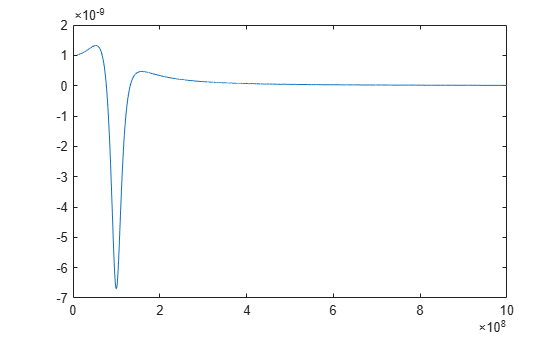# groupdelay

Group delay of S-parameter object or RF filter object or RF Toolbox circuit object

## Syntax

``gd = groupdelay(sparamobj)``
``gd = groupdelay(sparamobj,i,j)``
``gd = groupdelay(rfobj,freq)``
``gd = groupdelay(rfobj,freq,Name,Value)``

## Description

example

````gd = groupdelay(sparamobj)` calculates the group delay of an S-parameter object at the frequencies specified in the S-parameter object file. `sparamobj` can be an S-parameters object or an `nport` object.```
````gd = groupdelay(sparamobj,i,j)` calculates the group delay of a specific `Sij`. If i, j are not specified, the group delay is calculated for `S21` for two-port objects and `S11` for non-two-port objects.```

example

````gd = groupdelay(rfobj,freq)` calculates the group delay of an RF Toolbox™ network object, `rfobj`, at the specified frequencies. ```
````gd = groupdelay(rfobj,freq,Name,Value)` calculates the group delay using additional options specified by one or more name-value pair arguments. Example: ```gd = groupdelay (filter, frequency, 'Aperture', 50)``````

## Examples

collapse all

Calculate and plot the group delay of an RLC notch filter at the frequency range of `10``1000 `MHz.

```filt = circuit('notch'); add(filt,[1 2],resistor(200)); add(filt,[1 2],inductor(100e-9)); add(filt,[1 2],capacitor(25e-12)); setports(filt,[1 0],[2 0]); freq = 10e6:10e4:1000e6; gd = groupdelay(filt,freq); figure plot(freq,gd)```Find and plot the group delay from the specified Touchstone® file.

```S = sparameters('defaultbandpass.s2p'); freq = S.Frequencies; gd = groupdelay(S,freq); figure plot(freq,gd)```Calculate and plot the group delay of an RF Filter object at the frequency range of `0.5 - 3.5` GHz.

```r = rffilter('FilterType','Butterworth','ResponseType',"Bandpass"); freq = linspace(0.5e9,3.5e9,1001); gd = groupdelay(r,freq); plot(freq/1e9,gd) xlabel('Frequency (GHz)'); ylabel('Group delay (seconds)');```Create a two-wire transmission line using these specifications:

• Dielectric - air

• Thickness of dielectric or separation - 1.088 mm

• Permittivity or `EpsilonR` - 1.0054

`twowiretxline = txlineTwoWire('Radius',0.5e-3,'EpsilonR',1.0054,'Separation',1.088e-3);`

Calculate the noise figure and the group delay of the transmission line at 2.5 GHz.

`nf = noisefigure(twowiretxline,2.5e9)`
```nf = 0 ```
`gd = groupdelay(twowiretxline,2.5e9)`
```gd = 3.3446e-11 ```

## Input Arguments

collapse all

S-parameter object. The function uses the data in the object to calculate the group delay.

Example: ```sparamobj = sparameters('defaultbandpass.s2p')```

RF object, specified as one of the following:

 Circuit object `circuit` RF Filter object `rffilter` and `lcladder`. Transmission line objects Series and Shunt RLC objects `seriesRLC`, and `shuntRLC` RF Network objects For complete list of RF network objects see, RF Network Parameter Objects.

Frequencies at which group delay is calculated, specified as a vector of positive real numbers.

Port numbers of S-parameter object or RF object, specified as scalar integers.

Example: `S12`

### Name-Value Arguments

Specify optional pairs of arguments as `Name1=Value1,...,NameN=ValueN`, where `Name` is the argument name and `Value` is the corresponding value. Name-value arguments must appear after other arguments, but the order of the pairs does not matter.

Before R2021a, use commas to separate each name and value, and enclose `Name` in quotes.

Example: ```gd = groupdelay (filter, frequency, 'Aperture', 50)```

Width of two frequency points, specified as the comma-separated pair consisting of `'Aperture'` and a real, positive, numerics scalar or vector.

Example: `'Aperture',50`

Data Types: `double`

Impedance of S-parameters, specified as the comma-separated pair consisting of `'Impedance'` and a real positive numeric scalar. The default impedance values for different objects are:

• `50` — LC ladder and circuit objects

• `obj.impedance` — S-parameter objects

• `obj.networkdata.impedance` — N-port objects

Example: `50`

Data Types: `double`

## Output Arguments

collapse all

Group delay, returned as a numeric scalar in seconds.

## Version History

Introduced in R2015b

expand all